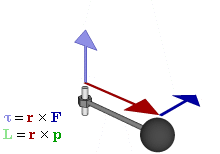## FANDOM

162 PagesAnimation showing the relationship between force (F), torque (τ), linear momentum (p), angular momentum (L), and position (r) of arotating object.

The angular momentum (symbol L) of an object is its angular velocity (ω, the rate at which the angle is changing) times the moment of inertia (I, equal to the mass of the object multiplied by its distance from the pivot point squared). In mathematical terms,

$\mathbf{L} = I \omega \ = \omega m r^2$

It is also equal to the cross product of the position vector (r) and linear momentum (p), and is therefore a pseudovector:

$\mathbf{L}=\mathbf{r}\times\mathbf{p}$

If the net torque on a rotational system is zero, angular momentum will be conserved.

Community content is available under CC-BY-SA unless otherwise noted.